# Kernel Density Estimation¶

by Suraj Rampure (suraj.rampure@berkeley.edu), for Data 100, Spring 2019

Click here to play with the interactive version of this notebook.

This notebook aims to explain Kernel Density Estimation.

Before we begin, let's import the necessary packages and implement a few important functions.

In :
import numpy as np
import matplotlib.pyplot as plt
%matplotlib inline

In :
def gaussian(x, z, a):
# Gaussian kernel
return (1/np.sqrt(2*np.pi*a**2)) * np.e ** (-(x - z)**2 / (2 * a**2))

def boxcar(x, z, a):
# Boxcar kernel
if np.abs(x - z) <= a/2:
return 1/a
return 0

In :
def create_kde(kernel, pts, a):
# Takes in a kernel, set of points, and alpha
# Returns the KDE as a function
def f(x):
output = 0
for pt in pts:
output += kernel(x, pt, a)
return output / len(pts) # Normalization factor
return f

def plot_kde(kernel, pts, a):
# Calls create_kde and plots the corresponding KDE
f = create_kde(kernel, pts, a)
x = np.linspace(min(pts) - 5, max(pts) + 5, 1000)
y = [f(xi) for xi in x]
plt.plot(x, y);

def plot_separate_kernels(kernel, pts, a):
# Plots individual kernels, which are then summed to create the KDE
x = np.linspace(min(pts) - 5, max(pts) + 5, 1000)
for pt in pts:
y = [kernel(xi, pt, a) for xi in x]
plt.plot(x, y)

plt.show();


Before we talk about kernel density estimation, we need to talk about a larger problem – density estimation. Currently, if we want to visualize the distribution of a set of values that we're given, we tend to use a histogram.

In :
points = [3, 4, 9, 12, 13]

In :
plt.hist(points);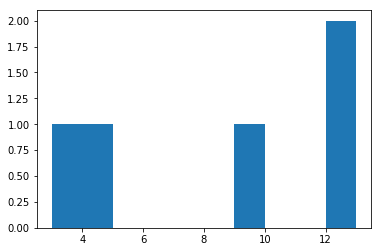Sure, this gives us an estimate of the distribution that our dataset comes from. But, histograms aren't smooth, and are hard to generalize into higher dimensions.

Kernel density estimation (KDE) attempts to create a smooth function that estimates the underlying distribution (and more specifically, the probability distribution that our values are drawn from). To determine a KDe, we first center a kernel at every single one of our points, where a kernel is a probability distribution on its own (i.e. it is a function whose area integrates to 1). We then take the sum of each of these individual kernels to yield the density estimate. The choice of kernel is up to us; typically we will choose to use the Gaussian kernel.

First, let's look at individual Gaussian kernels for our set of points, $S = \{3, 4, 9, 12, 13\}$ (ignore the parameter $a$ for now):

In :
plt.title('Separate Kernels with alpha = 1 (n = 5)');
plot_separate_kernels(gaussian, points, a = 1)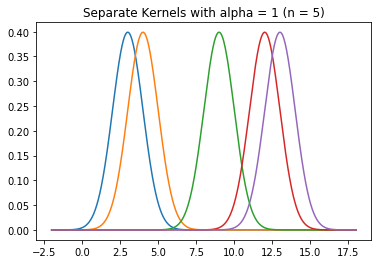Now, let's determine the function that results from adding each of these separate functions together (and dividing the result by 5, to ensure it remains a valid probability density function):

In :
plot_kde(gaussian, points, a = 1)
plt.title('KDE with Gaussian Kernel and alpha = 1 (n = 5)');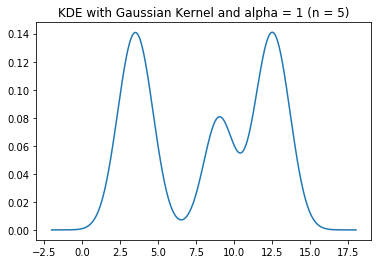This resulting curve, for our purposes, does the same job as a histogram.

Before going any further, let's look at the definition of the Gaussian kernel:

$$K_{\alpha}(x, z) = \frac{1}{\sqrt{2 \pi \alpha^2}} e^{-\frac{(x - z)^2}{2\alpha^2}}$$

This may look familiar – this is precisely the probability density function of a Gaussian (normal) random variable with mean $z$ and standard deviation $\alpha$.

Suppose our input values are $z_1, z_2, ..., z_n$. Then, any one individual kernel will be of the form $\frac{1}{\sqrt{2 \pi \alpha^2}} e^{-\frac{(x - z_i)^2}{2\alpha^2}}$. The entire KDE, then, will have the equation

$$K_\alpha(x) = \sum_{i = 1}^n K_{\alpha}(x, z) = \sum_{i = 1}^n \frac{1}{\sqrt{2 \pi \alpha^2}} e^{-\frac{(x - z_i)^2}{2\alpha^2}}$$

Notice, $\alpha$ is the standard deviation of individual kernels. If the variance (i.e. the square of the standard deviation) of a Gaussian curve is large, it means that the distribution is very spread out, and the peak is not very tall. In contrast, if the variance is relatively small, most values will be concentrated around the mean, and there will be sharper peak. A good way to think about $\alpha$ is in relation to the number of bins we use in a histogram. A large $\alpha$ corresponds to using fewer bins, and vice versa.

Let's look at KDE plots of a different dataset using a few different values of $\alpha$. To illustrate the point, we'll also look at two histograms with different bin sizes.

In :
points_random = [100 * np.random.random() for i in range(100)]

In :
plt.hist(points_random, bins=10)
plt.title('Histogram with 10 Bins (n = 100)');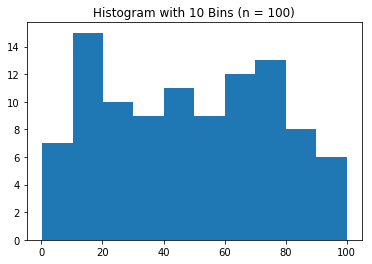In :
plt.hist(points_random, bins=50)
plt.title('Histogram with 50 Bins (n = 100)');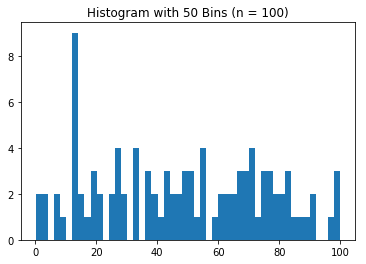In :
plot_kde(gaussian, points_random, a = 0.1)
plt.title('KDE with Gaussian Kernel and alpha = 0.1 (n = 100)');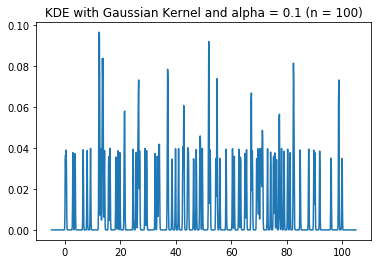In :
plot_kde(gaussian, points_random, a = 1)
plt.title('KDE with Gaussian Kernel and alpha = 1 (n = 100)');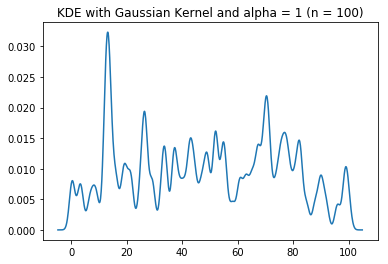In :
plot_kde(gaussian, points_random, a = 4)
plt.title('KDE with Gaussian Kernel and alpha = 4 (n = 100)');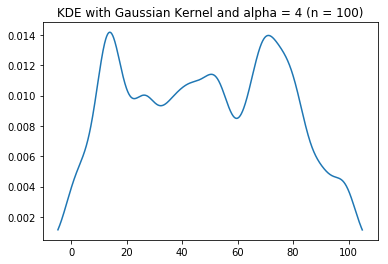In :
plot_kde(gaussian, points_random, a = 10)
plt.title('KDE with Gaussian Kernel and alpha = 10 (n = 100)');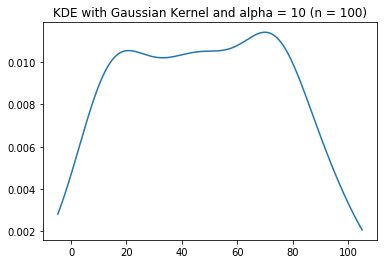The larger $\alpha$ is, the more smooth our resulting curve is. There are benefits and drawbacks to both a large $\alpha$ and small $\alpha$. With a smaller $\alpha$, we see more detail from the dataset that was used to generate the estimate, but the resulting estimate may not necessarily generalize well if we were to use this function to do any sort of prediction. With a larger $\alpha$, the curve looks more general and smooth, but we mask some of the structure and lose some detail from the dataset we used to create the estimate.

Lastly, let's look at the Boxcar kernel. The boxcar kernel has the distribution

$$K_{\alpha}(x, z) = \begin {cases} \frac{1}{\alpha}, \: \: \: |x - z| \leq \frac{\alpha}{2}\\ 0, \: \: \: \text{else} \end{cases}$$

Notice, the Gaussian distribution assigns non-zero probability to any real number input $x$, whereas the boxcar kernel only assigns probability within a region of $\frac{\alpha}{2}$ from the observation point ($z$). We can also verify that the boxcar kernel is a probability distribution with area 1, as it takes on the shape of a rectangle with height $\frac{1}{\alpha}$ and width $\alpha$.

In :
plt.title('Individual Boxcar Kernel with alpha = 0.5 (n = 1)');
plot_separate_kernels(boxcar, , a = 0.5)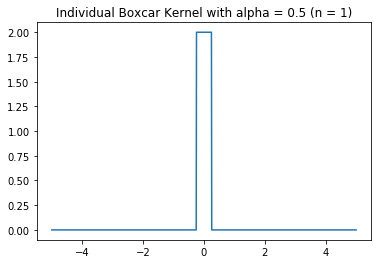Now, let's look at how the boxcar kernel behaves on the same data we looked at before.

In :
plot_kde(boxcar, points, a = 1)
plt.title('KDE with Boxcar Kernel and alpha = 1 (n = 5)');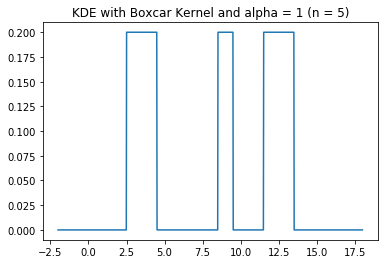In :
plot_kde(boxcar, points_random, a = 0.1)
plt.title('KDE with Boxcar Kernel and alpha = 0.1 (n = 100)');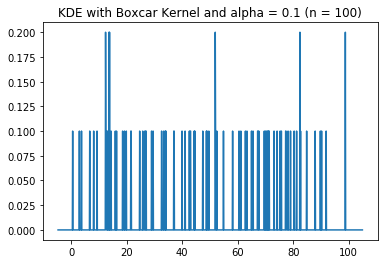In :
plot_kde(boxcar, points_random, a = 1)
plt.title('KDE with Boxcar Kernel and alpha = 1 (n = 100)');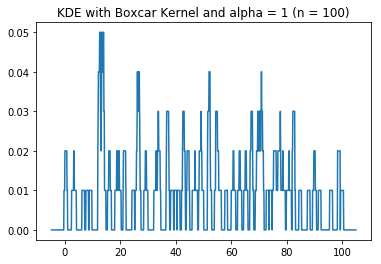In :
plot_kde(boxcar, points_random, a = 4)
plt.title('KDE with Boxcar Kernel and alpha = 4 (n = 100)');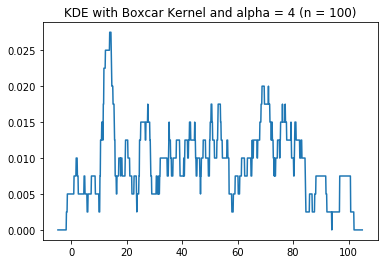In :
plot_kde(boxcar, points_random, a = 10)
plt.title('KDE with Boxcar Kernel and alpha = 10 (n = 100)');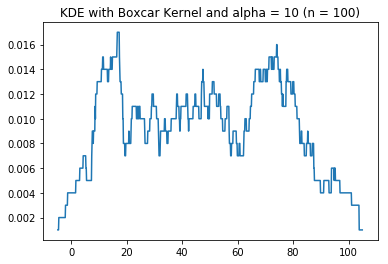Feel free to reach out if you have any questions!